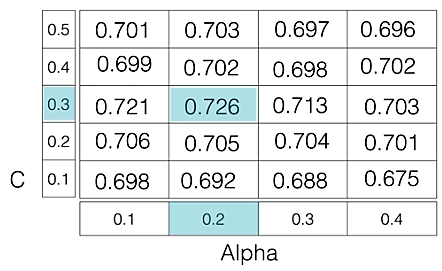# Hyperparameter tuning

A Machine Learning model is defined as a mathematical model with a number of parameters that need to be learned from the data. By training a model with existing data, we are able to fit the model parameters.
However, there is another kind of parameter, known as Hyperparameters, that cannot be directly learned from the regular training process. They are usually fixed before the actual training process begins. These parameters express important properties of the model such as its complexity or how fast it should learn.

Some examples of model hyperparameters include:

1. The penalty in Logistic Regression Classifier i.e. L1 or L2 regularization
2. The learning rate for training a neural network.
3. The C and sigma hyperparameters for support vector machines.
4. The k in k-nearest neighbors.

Models can have many hyperparameters and finding the best combination of parameters can be treated as a search problem. The two best strategies for Hyperparameter tuning are:

GridSearchCV
In GridSearchCV approach, the machine learning model is evaluated for a range of hyperparameter values. This approach is called GridSearchCV, because it searches for the best set of hyperparameters from a grid of hyperparameters values.

For example, if we want to set two hyperparameters C and Alpha of the Logistic Regression Classifier model, with different sets of values. The grid search technique will construct many versions of the model with all possible combinations of hyperparameters and will return the best one.

As in the image, for C = [0.1, 0.2, 0.3, 0.4, 0.5] and Alpha = [0.1, 0.2, 0.3, 0.4]. For a combination of C=0.3 and Alpha=0.2, the performance score comes out to be 0.726(Highest), therefore it is selected.The following code illustrates how to use GridSearchCV

## Python3

 `# Necessary imports` `from` `sklearn.linear_model ``import` `LogisticRegression` `from` `sklearn.model_selection ``import` `GridSearchCV`   `# Creating the hyperparameter grid` `c_space ``=` `np.logspace(``-``5``, ``8``, ``15``)` `param_grid ``=` `{``'C'``: c_space}`   `# Instantiating logistic regression classifier` `logreg ``=` `LogisticRegression()`   `# Instantiating the GridSearchCV object` `logreg_cv ``=` `GridSearchCV(logreg, param_grid, cv ``=` `5``)`   `logreg_cv.fit(X, y)`   `# Print the tuned parameters and score` `print``("Tuned Logistic Regression Parameters: {}".``format``(logreg_cv.best_params_)) ` `print``("Best score ``is` `{}".``format``(logreg_cv.best_score_))`

Output:

Tuned Logistic Regression Parameters: {‘C’: 3.7275937203149381} Best score is 0.7708333333333334

Drawback: GridSearchCV will go through all the intermediate combinations of hyperparameters which makes grid search computationally very expensive.

RandomizedSearchCV
RandomizedSearchCV solves the drawbacks of GridSearchCV, as it goes through only a fixed number of hyperparameter settings. It moves within the grid in a random fashion to find the best set of hyperparameters. This approach reduces unnecessary computation.

The following code illustrates how to use RandomizedSearchCV

## Python3

 `# Necessary imports` `from` `scipy.stats ``import` `randint` `from` `sklearn.tree ``import` `DecisionTreeClassifier` `from` `sklearn.model_selection ``import` `RandomizedSearchCV`   `# Creating the hyperparameter grid ` `param_dist ``=` `{"max_depth": [``3``, ``None``],` `              ``"max_features": randint(``1``, ``9``),` `              ``"min_samples_leaf": randint(``1``, ``9``),` `              ``"criterion": ["gini", "entropy"]}`   `# Instantiating Decision Tree classifier` `tree ``=` `DecisionTreeClassifier()`   `# Instantiating RandomizedSearchCV object` `tree_cv ``=` `RandomizedSearchCV(tree, param_dist, cv ``=` `5``)`   `tree_cv.fit(X, y)`   `# Print the tuned parameters and score` `print``("Tuned Decision Tree Parameters: {}".``format``(tree_cv.best_params_))` `print``("Best score ``is` `{}".``format``(tree_cv.best_score_))`

Output:

Tuned Decision Tree Parameters: {‘min_samples_leaf’: 5, ‘max_depth’: 3, ‘max_features’: 5, ‘criterion’: ‘gini’} Best score is 0.7265625

Whether you're preparing for your first job interview or aiming to upskill in this ever-evolving tech landscape, GeeksforGeeks Courses are your key to success. We provide top-quality content at affordable prices, all geared towards accelerating your growth in a time-bound manner. Join the millions we've already empowered, and we're here to do the same for you. Don't miss out - check it out now!

Previous
Next